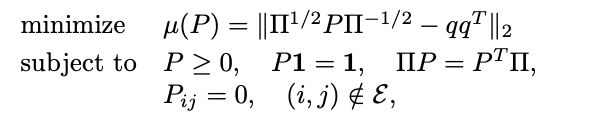# CVX giving ‘Solved’ outcome not satisfying the constraints

Hello,

I’m trying to solve an optimization problem, but sometimes (it depends on the setup), even if the problem is ‘Solved’, the constraints are violated (of a non-negligible value for my purpose).

The constraint is not ignored: The results give very small number for the variable with constraint == 0.

Do you know what can be the possible reason for it?

The problem I am trying to solve iswhere \mathcal{E} is the set where a matrix A has zero entries.
A = np.array([[1,1,0,1,1],[1,1,1,0,1],[0,1,1,1,1],[1,0,1,1,1],[1,1,1,1,1]])
L = np.array([4,5,7,6,11])
n = A.shape
P = np.zeros((n,n))
sumL = (A - np.identity(n)).dot(L)
pi = L/np.sum(L)
pi_sqrt = np.sqrt(pi)
Q = np.outer(pi_sqrt, pi_sqrt.T)
PI_sqrt = np.diag(pi_sqrt)
PI_sqrt_inv = np.diag(1/pi_sqrt)
X = cp.Variable((n,n), symmetric=False)
s = cp.Variable()
one = np.ones(n)
iden = np.identity(n)
zero_index = []
for i in range(n):
for j in range(n):
if A[i,j] == 0:
zero_index.append([i,j])
zero_indeces = tuple(zip(zero_index))
zero_values = np.zeros(len(zero_index))
constraints = [cp.vec(X) >= 0]
constraints += [PI_sqrt@X@PI_sqrt_inv - Q + s
iden >> 0]
constraints += [0 >> PI_sqrt@X@PI_sqrt_inv - Q - s*iden]
constraints += [X@one == one]
constraints += [PI_sqrt@X == X.T@PI_sqrt]
constraints += [X[zero_indeces] == 0]
prob = cp.Problem(cp.Minimize(s), constraints)
prob.solve(solver = cp.SCS)
P = X.value

And the outcome gives:
optimal
0.23075128629982344
[[ 2.00945263e-01 2.24474692e-01 -1.60803788e-11 2.43695617e-01
3.30884428e-01]
[ 2.00776268e-01 2.22688940e-01 2.59026263e-01 -1.86612685e-11
3.17508528e-01]
[-1.73584394e-11 2.18917148e-01 2.50375526e-01 2.35000873e-01
2.95706452e-01]
[ 1.98976638e-01 -1.72719877e-11 2.53829954e-01 2.37736614e-01
3.09456795e-01]
[ 1.99530818e-01 2.14064206e-01 2.35892144e-01 2.28548990e-01
1.21963842e-01]]

I don’t know what the individual numbers in the output are supposed to represent. But numbers such as -1.6e-11 and -1.7e-11 are not distinguishable from 0 for solver purposes; and due to feasibility tolerance, they would not be considered to be in violation of any constraints that they be nonnegative or that they equal zero.

In any event, this is the CVX (MATLAB) forum, not CVXPY (Python). Please seek support in the appropriate CVXPY support venues.

1 Like